# A country has GDP per capita equal to \$5,000. If the country's GDP per capita increases...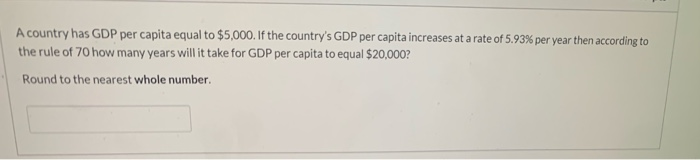A country has GDP per capita equal to \$5,000. If the country's GDP per capita increases at a rate of 5.93% per year then according to the rule of 70 how many years will it take for GDP per capita to equal \$20,000? Round to the nearest whole number.

According to the rule of 70, we can find out the no. of years in which a perticular sum will double by dividing 70 by the growth rate.

So, here \$5,000 would bexomeibecome \$10,000 in 70/5.93 = 11.80 years.

Similarly, \$10,000 would become \$20,000 in 70/5.93 = 11.80 years.

So, total number of years required for \$5,000 to become \$10,000 = 11.80 +11.80 = 23.60 years.

##### Add Answer of: A country has GDP per capita equal to \$5,000. If the country's GDP per capita increases...
Similar Homework Help Questions
• ### A country has GDP per capita equal to \$5,000. If the country’s GDP per capita increases...

A country has GDP per capita equal to \$5,000. If the country’s GDP per capita increases at a rate of 3.60% per year then according to the rule of 70 how many years will it take for GDP per capita to equal \$20,000? Round to the nearest whole number.

• ### QUESTION 41 10 According to the rule of 70, if a country's real GDP per capita...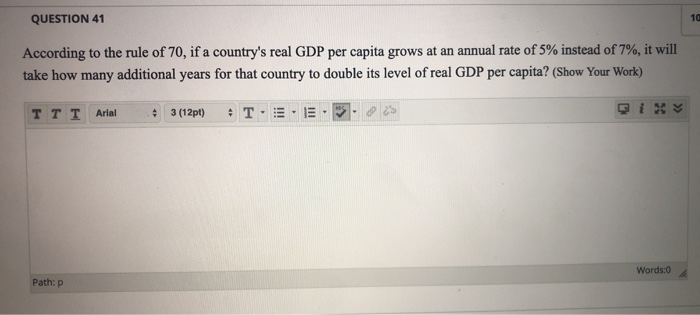QUESTION 41 10 According to the rule of 70, if a country's real GDP per capita grows at an annual rate of 5% instead of 7%, it will take how many additional years for that country to double its level of real GDP per capita? (Show Your Work) TTT Arial • 3 (12pt) + T. Words:0 Path: P

• ### 2. Country A’s current GDP is \$1,000,000. It is growing at the rate of 2% per...

2. Country A’s current GDP is \$1,000,000. It is growing at the rate of 2% per year. It has a current population of 2,500 which is growing at 3% a year. (a) Using the rule of 70, how long will it be before Country A’s GDP doubles (round off to the nearest value)? What will it’s per-capita GDP be in that year? (b) Using the rule of 70, how long will it be before Country A’s population doubles (round off...

• ### Country A starts with real GDP per capita equal to \$40,000 and Country B starts with...

Country A starts with real GDP per capita equal to \$40,000 and Country B starts with real GDP per capita equal to \$2,000. Today the RGDP per capita in A is ___ times the value in B. Country A is growing at a rate of 3.5% per year and Country B is growing at a rate of 7% per year. Assume these growth rates do not change. Country A will double its RGDP per capita in _____ years and country...

• ### Assume that a "leader country has real GDP per capita of \$40,000, whereas a "follower country"...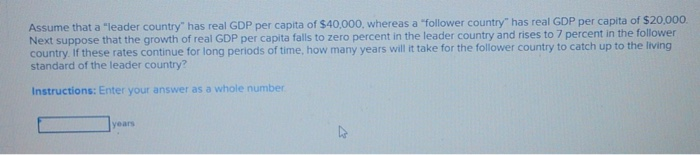Assume that a "leader country has real GDP per capita of \$40,000, whereas a "follower country" has real GDP per capita of \$20,000. Next suppose that the growth of real GDP per capita falls to zero percent in the leader country and rises to 7 percent in the follower country. If these rates continue for long periods of time, how many years will it take for the follower country to catch up to the living standard of the leader country?...

• ### Two countries decide to specialize in producing certain goods to export to other countries, and in...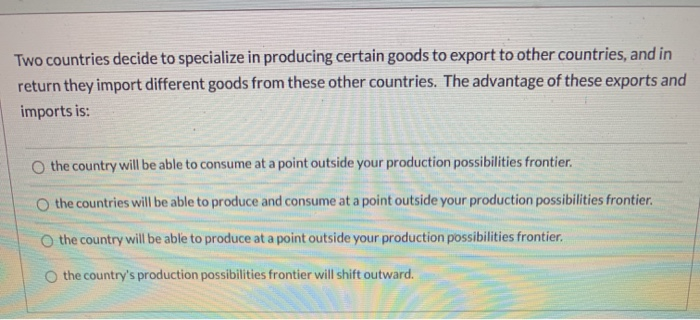Two countries decide to specialize in producing certain goods to export to other countries, and in return they import different goods from these other countries. The advantage of these exports and imports is: the country will be able to consume at a point outside your production possibilities frontier the countries will be able to produce and consume at a point outside your production possibilities frontier. the country will be able to produce at a point outside your production possibilities frontier....

• ### GDP per capita in Latvia? GDP per capita in the country? GDP growth in Latvia%? Growth...

GDP per capita in Latvia? GDP per capita in the country? GDP growth in Latvia%? Growth of GDP per capita in Latvia%? GDP growth in the country%? Growth of GDP per capita in the country%? Number of years when Latvia achieves the same level (based on GDP growth)? Number of years when Latvia achieves the same level ( based on growth of GDP per capita)? The number must be written as% but without the simbol%

• ### According to the "Rule of 70", how many years will it take for real GDP per capita to double when the growth rate of re...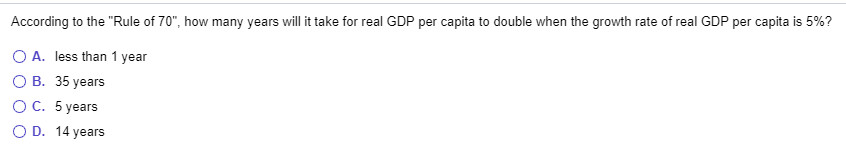According to the "Rule of 70", how many years will it take for real GDP per capita to double when the growth rate of real GDP per capita is 5%? O A. less than 1 year OB. 35 years O C. 5 years OD. 14 years

• ### Question 3 2.5 pts Question 2: the rule of 70 Country A starts with real GDP...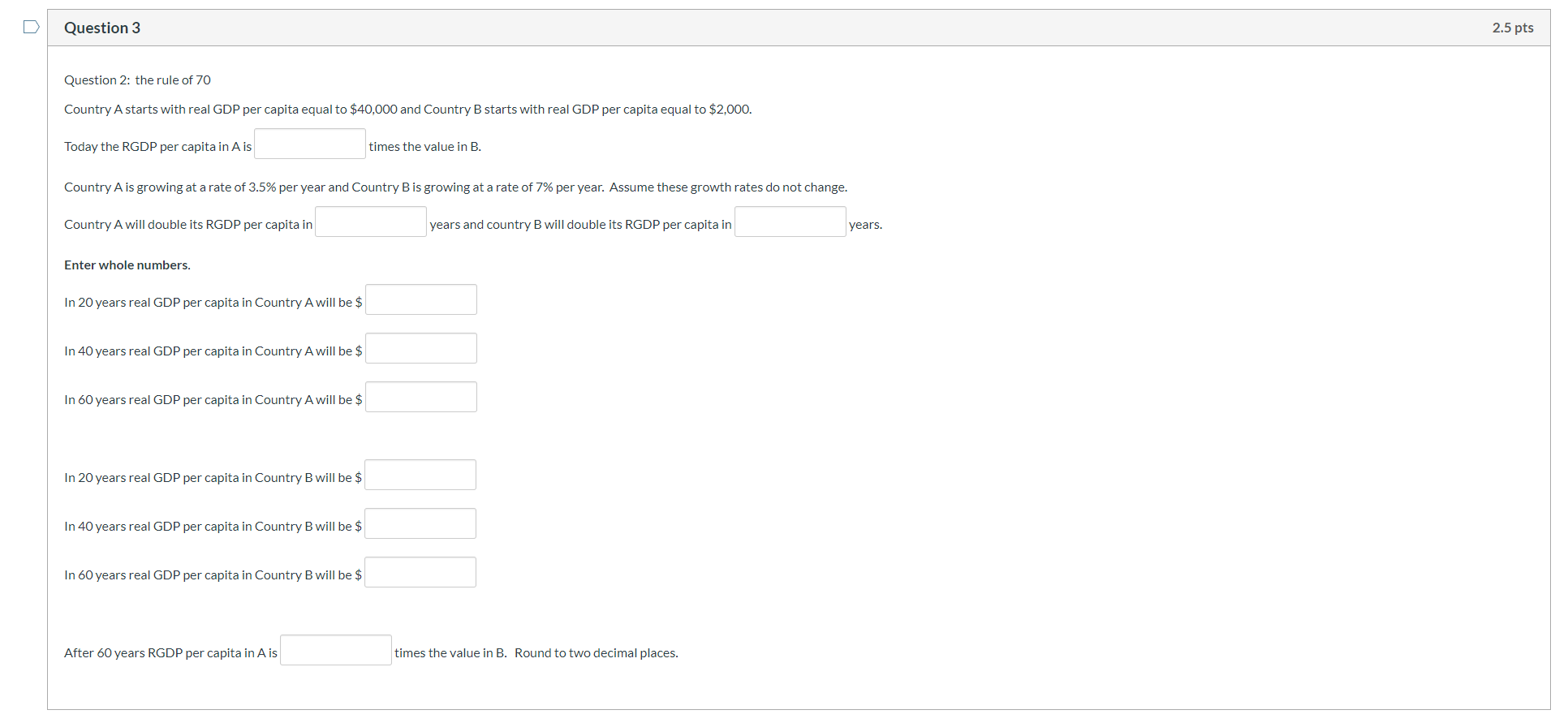Question 3 2.5 pts Question 2: the rule of 70 Country A starts with real GDP per capita equal to \$40,000 and Country B starts with real GDP per capita equal to \$2,000. Today the RGDP per capita in A is times the value in B. Country A is growing at a rate of 3.5% per year and Country B is growing at a rate of 7% per year. Assume these growth rates do not change. Country A will double...

• ### Need help, please show work Country A has a per capita income of \$4,000 per year...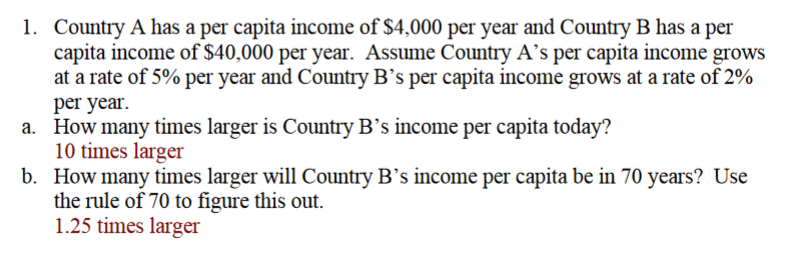Need help, please show work Country A has a per capita income of \$4,000 per year and Country B has a per capita income of S40.000 per year. Assume Country A's per capita income grows at a rate of 5% per year and Country B's per capita income grows at a rate of 290 per year How many times larger is Country B's income per capita today? 10 times larger How many times larger will Country B's income per capita...

Free Homework App